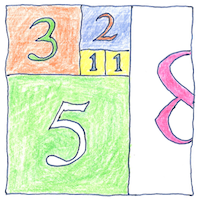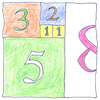# 1202 Number system

## The book of science

Tom Sharp

 Leonardo Pisano Bigollo (Fibonacci) mathematics## Number system

• In Liber Abaci, Leonardo Pisano Bigollo,
• known today as Fibonacci, the son of Bonacci,
• popularized the use of the nine numerals,
• the zero sign, and the concept of place value.
• In this book (the “Book of Calculation”),
• Fibonacci showed how to solve business problems—
• conversions of currency and measurements,
• and calculations of profit and interest.
• Fibonacci also showed how to use
• the number system for purely mathematical concepts—
• perfect numbers, primes,
• and series including the eponymous Fibonacci series.
• Finally Fibonacci described
• numeric and geometric approximations
• and irrational numbers such as the square root of two.

## Exchange

• The young Leonardo Pisano Bigollo
• was introduced to the Hindu-Arabic number system
• in Béjaïa, North Africa,
• where his father directed a customs house.
• exchanging both goods and ideas,
• Subsequently, on business, Leonardo traveled
• to Egypt, Syria, Greece, Sicily, and Provence
• and learned from the leading Arab mathematicians.

## Fibonacci sequence

• One and one is two; one and two is three;
• two and three is five and so forth;
• add the last two numbers to get the next
• to construct the Fibonacci sequence,
• describing how breeding rabbits increase
• and how leaves are arranged on a stem.
• Plus, the ratio of any two successive Fibonacci numbers
• approximates the golden number, phi,
• applicable to art, architecture,
• theories of beauty, or stock market analysis.
• Given a simple relation, a sequence
• of simple additions, a pattern emerges.

Fibonacci contributed to Europe’s adoption of arithmetical methods using the modern numeral system, as opposed to using counting boards (like the abacus) with Roman numerals.